﻿ 16074307 汪丽琼 数学建模实训 作业4 - 计算数学达人 - 专，学者，数值代数，微分方程数值解

### 16074307 汪丽琼 数学建模实训 作业4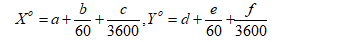1 犯罪地点地理剖面分布图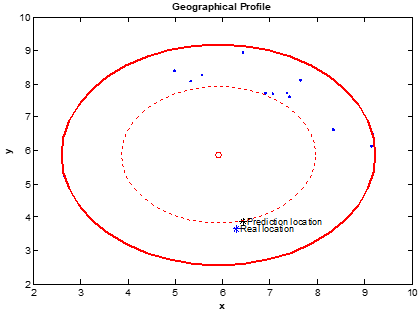2 预测犯罪地点地理剖面分布图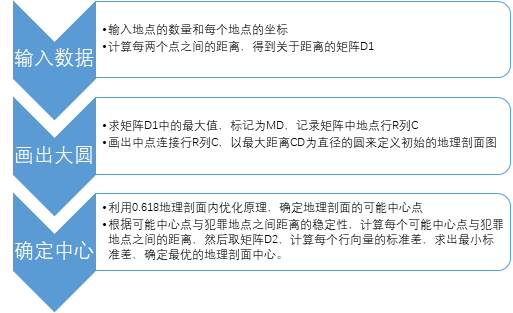3 算法流程图

预测模型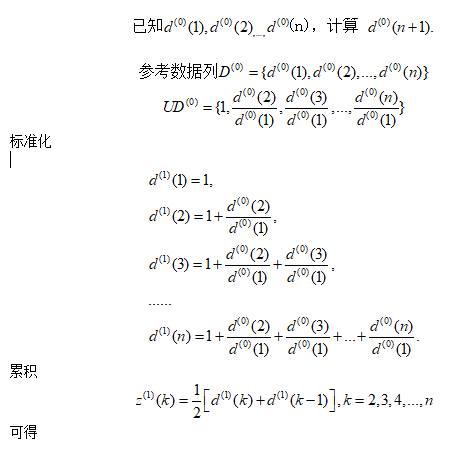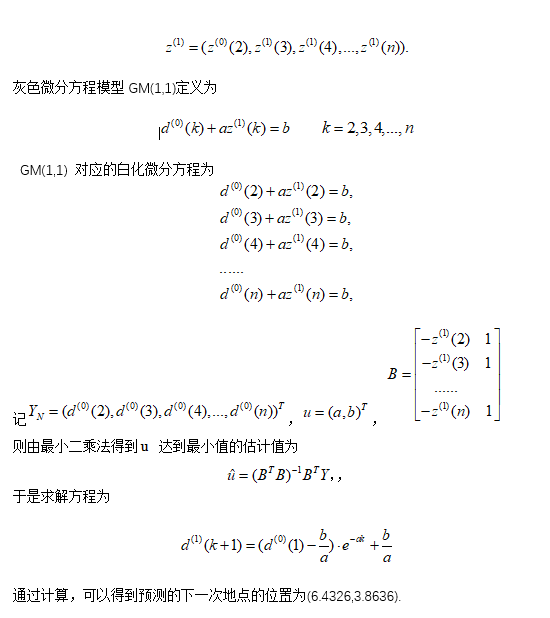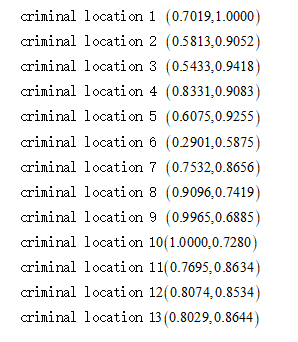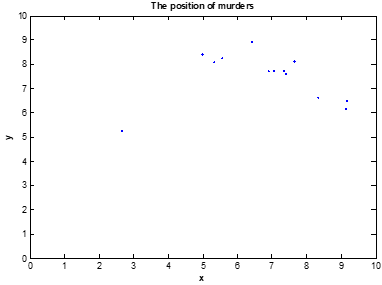4 犯罪地点分布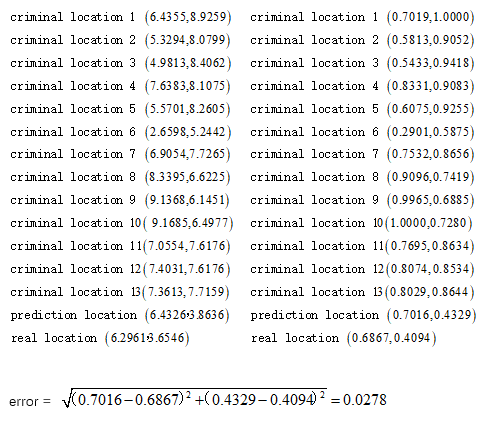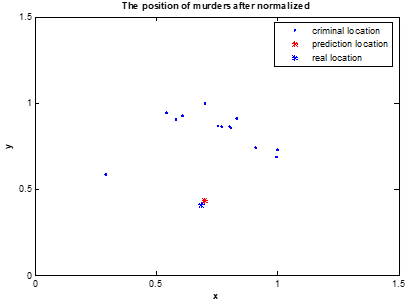5 标准化后的犯罪地点分布

Clear

clc

disp('----------------------  Geographical Profile  -----------------------');

n = input('Please input the number of points  ');

disp('Please input the coordinate of points');

distance = zeros(n-1,n-1);  t = 0;

for i = 1:n

fprintf('Point %d   n',i);

x(i) = input('X :  ');

y(i) = input('Y :  '); end

for j = 1:n

for k = j+1:n

distance(j,k) = sqrt((x(j)-x(k))^2+(y(j)-y(k))^2);;

end

end

max_dis = max(max(distance));

[p1 p2] = find(distance == max_dis);

max_cirx = (x(p1)+x(p2))/2;

max_ciry = (y(p1)+y(p2))/2;

rmax = max_dis/2;

theta = 0:pi/20:2*pi;

max_x = max_cirx + rmax*cos(theta);

max_y = max_ciry + rmax*sin(theta);

for z = 1:length(theta)

for k = 1:n

dis_cir(z,k) =

sqrt((max_x(z)-x(k))^2+(max_y(z)+rmax*0.382-y(k))^2);

end

end

for i=1:n

plot(x(i),y(i),'b.','MarkerSize',8);

hold on

end

plot(max_x,max_y,'r-','LineWidth',2);

xlabel('bfx');

ylabel('bfy'); axis([0 10 0 10]);

title('bfGeographical Profile');

for t = 1:length(theta)

dis_std(t) = std(dis_cir(t,:));

end

[mindis_std mark] = min(dis_std);

obj_x = max_cirx + rmax*cos(pi*mark/20);

obj_y = max_ciry + rmax*0.382 + rmax*sin(pi*mark/20);

plot(obj_x,obj_y,'k*','MarkerSize',8);

plot(max_cirx,max_ciry,'ro','MarkerSize',6); theta2 = 0:pi/20:2*pi;

small_cirx = max_cirx + rmax*0.618*cos(theta2);

small_ciry = max_ciry + rmax*0.618*sin(theta2);

plot(small_cirx,small_ciry,'r:');

hold off